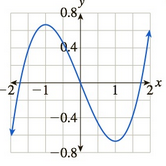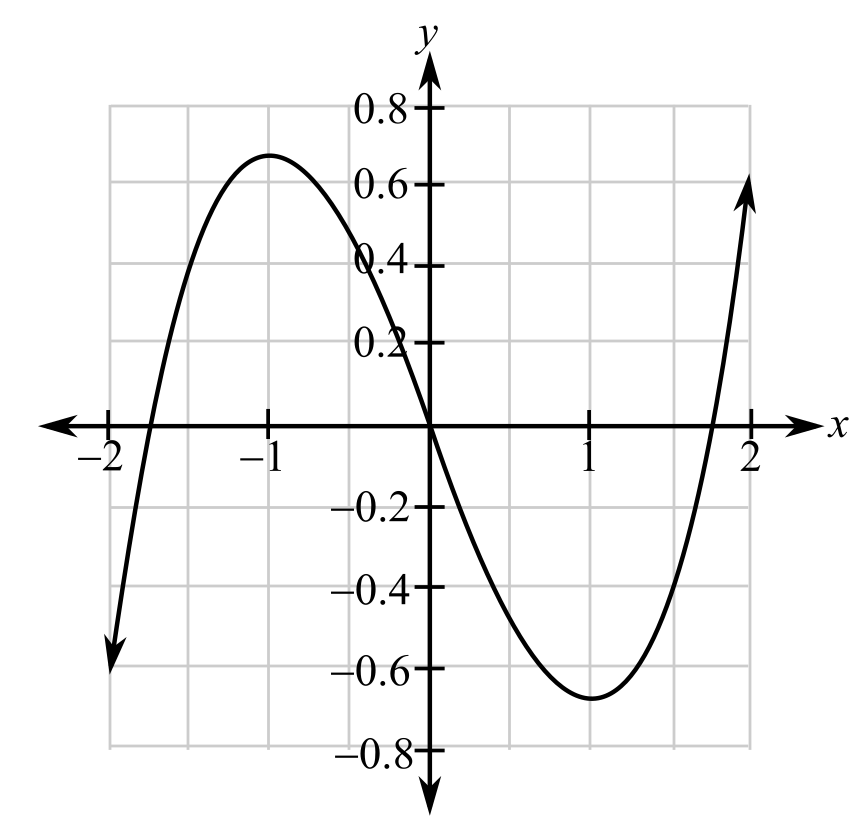Chapter 10.5, Problem 67EFinite Mathematics and Applied Cal...

7th Edition
Stefan Waner + 1 other
ISBN: 9781337274203

Solutions

Chapter
SectionFinite Mathematics and Applied Cal...

7th Edition
Stefan Waner + 1 other
ISBN: 9781337274203
Textbook Problem

In Exercises 65–68 the graph of a function is given. For which x in the range shown is the function increasing? For which x is the function decreasing? [HINT: See Quick Example 6.]To determine

The increasing and decreasing range of the function.

The provided graph is:Explanation

Given information:

The provided graph is:

When slope of the function is positive then it is an increasing function where as if the slope is negative then the function is decreasing.

From the graph one can observe that the curve rebounds at two points around (1,0.7) and (1,0.7)

Still sussing out bartleby?

Check out a sample textbook solution.

See a sample solution

The Solution to Your Study Problems

Bartleby provides explanations to thousands of textbook problems written by our experts, many with advanced degrees!

Get Started

perform the indicated operations and simplify each expression. 128. (x2 4)(x2 + 4)(2x + 8) (x2 + 8x 4)(4x3)

Applied Calculus for the Managerial, Life, and Social Sciences: A Brief Approach

Evaluate the improper integrals in Problems 31-34. 31.

Mathematical Applications for the Management, Life, and Social Sciences

True or False: The graph at the right is the graph of an even function.

Study Guide for Stewart's Single Variable Calculus: Early Transcendentals, 8th